# Searching Topics Sequential Search Binary Search Search Algorithms

• Slides: 22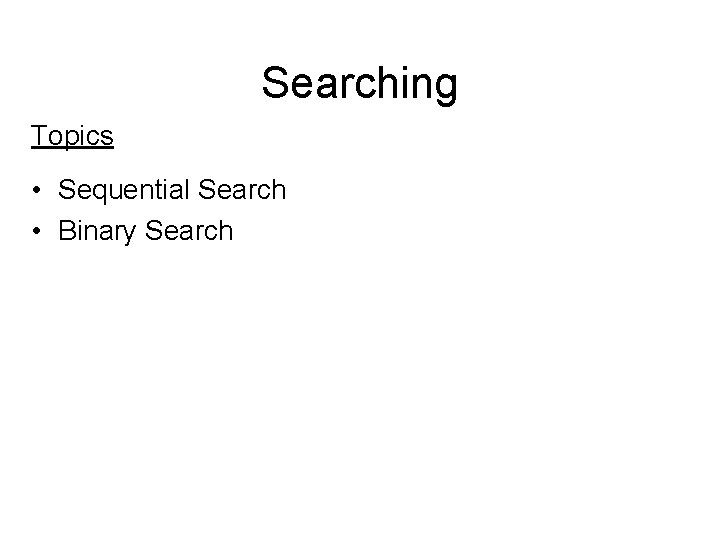Searching Topics • Sequential Search • Binary SearchSearch Algorithms The most important operation on a list is the search algorithm Using a search algorithm you can: – Determine whether a particular item is in the list – If the data are specially organized, find the location where a new item can be inserted – Find the location of an item to be deleted The search algorithm’s performance (search time) is crucial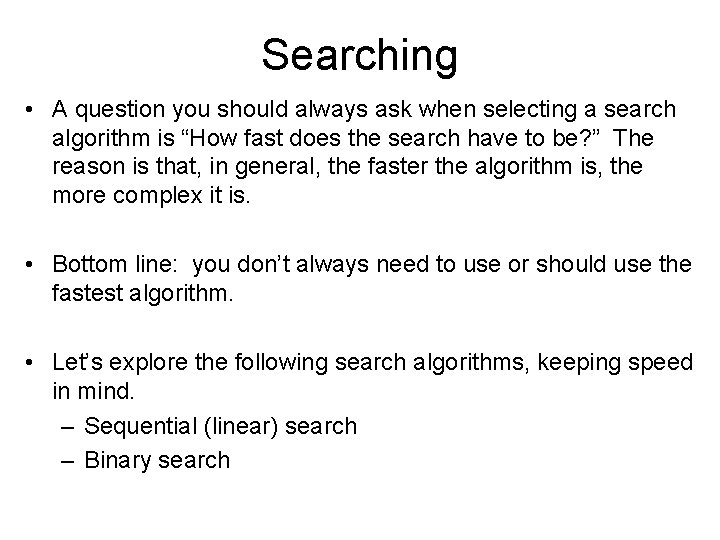Searching • A question you should always ask when selecting a search algorithm is “How fast does the search have to be? ” The reason is that, in general, the faster the algorithm is, the more complex it is. • Bottom line: you don’t always need to use or should use the fastest algorithm. • Let’s explore the following search algorithms, keeping speed in mind. – Sequential (linear) search – Binary search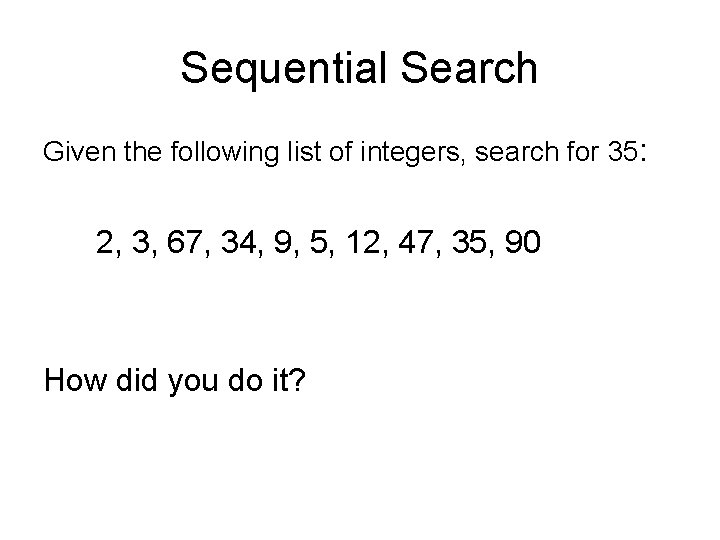Sequential Search Given the following list of integers, search for 35: 2, 3, 67, 34, 9, 5, 12, 47, 35, 90 How did you do it?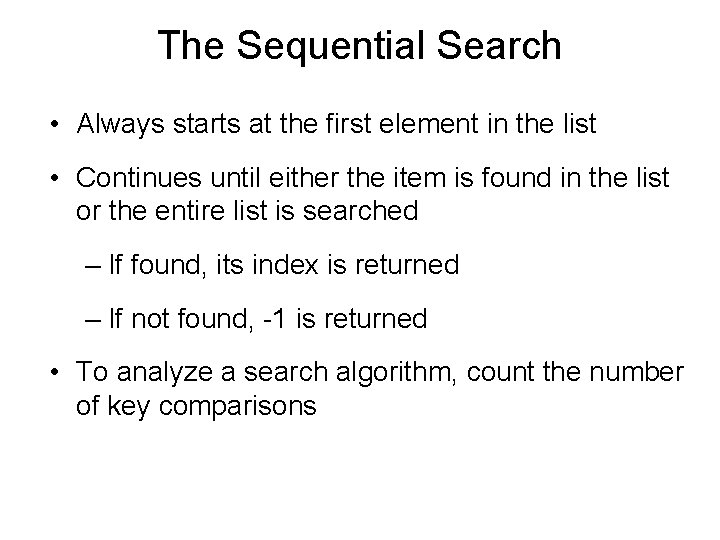The Sequential Search • Always starts at the first element in the list • Continues until either the item is found in the list or the entire list is searched – If found, its index is returned – If not found, -1 is returned • To analyze a search algorithm, count the number of key comparisons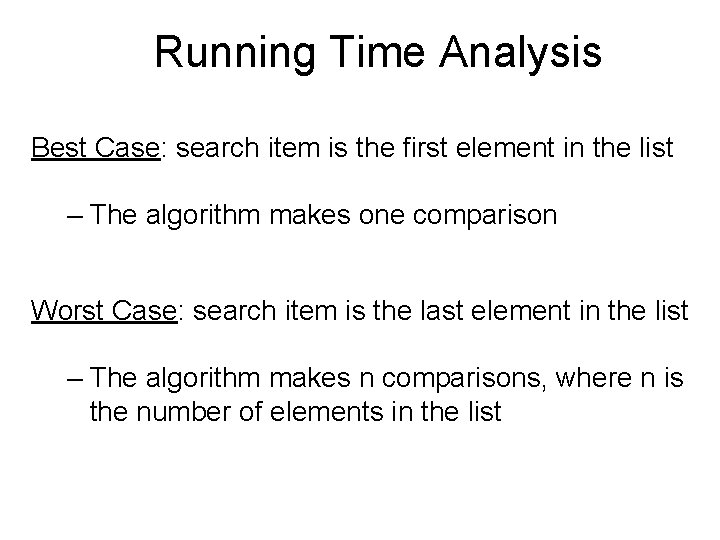Running Time Analysis Best Case: search item is the first element in the list – The algorithm makes one comparison Worst Case: search item is the last element in the list – The algorithm makes n comparisons, where n is the number of elements in the list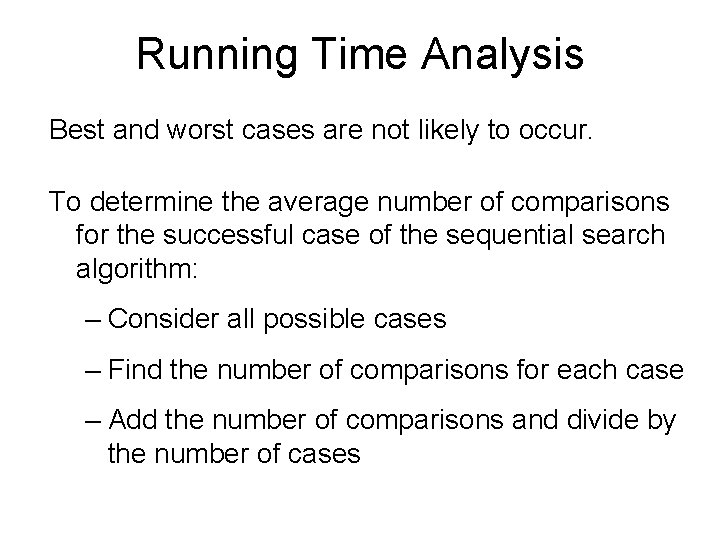Running Time Analysis Best and worst cases are not likely to occur. To determine the average number of comparisons for the successful case of the sequential search algorithm: – Consider all possible cases – Find the number of comparisons for each case – Add the number of comparisons and divide by the number of cases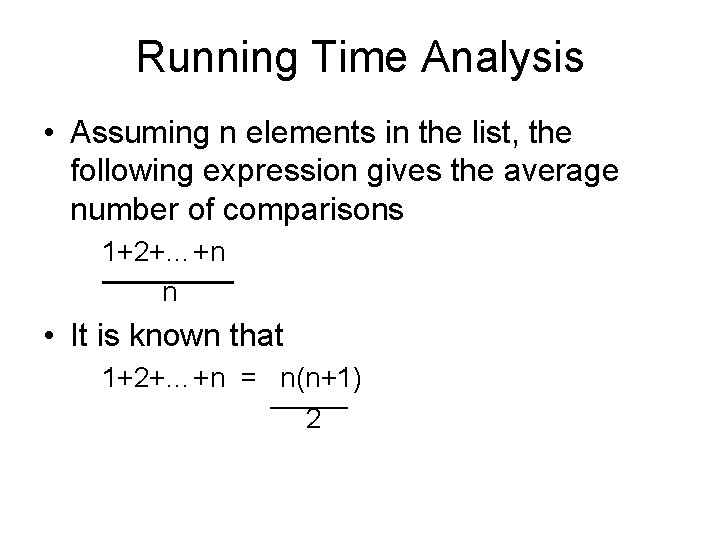Running Time Analysis • Assuming n elements in the list, the following expression gives the average number of comparisons 1+2+…+n n • It is known that 1+2+…+n = n(n+1) 2Running Time Analysis The average number of comparisons in the successful case is: 1+2+…+n = 1 n(n+1) n n 2 = n+1 2 On average the sequential search algorithm searches half the list Not good for large lists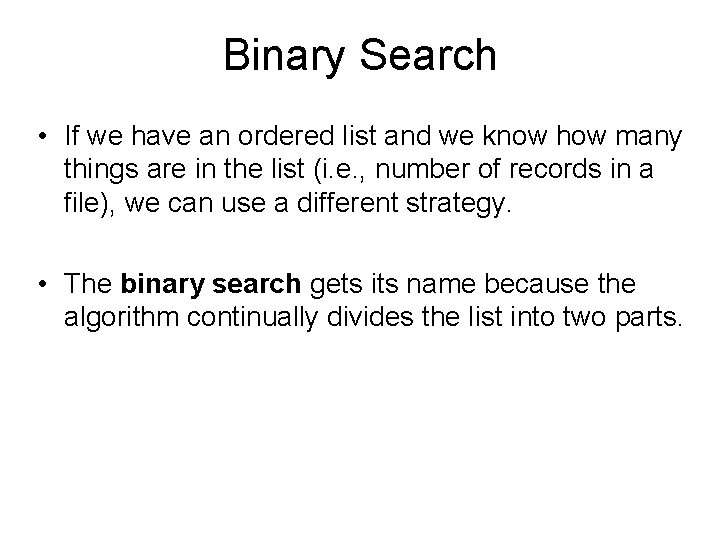Binary Search • If we have an ordered list and we know how many things are in the list (i. e. , number of records in a file), we can use a different strategy. • The binary search gets its name because the algorithm continually divides the list into two parts.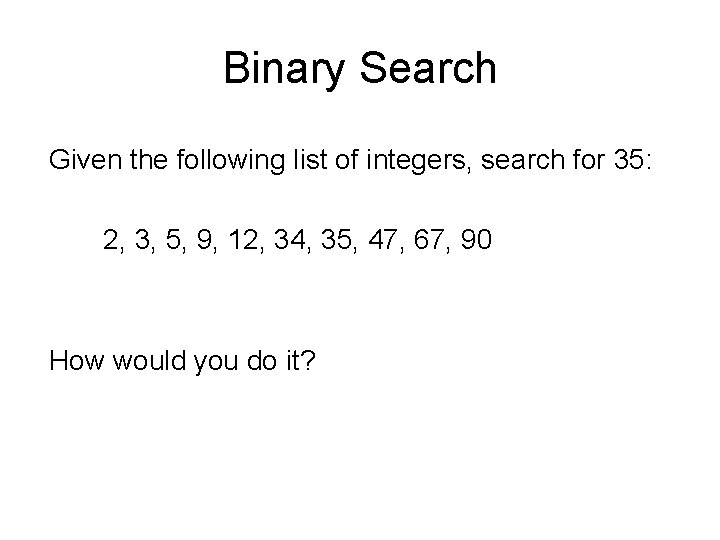Binary Search Given the following list of integers, search for 35: 2, 3, 5, 9, 12, 34, 35, 47, 67, 90 How would you do it?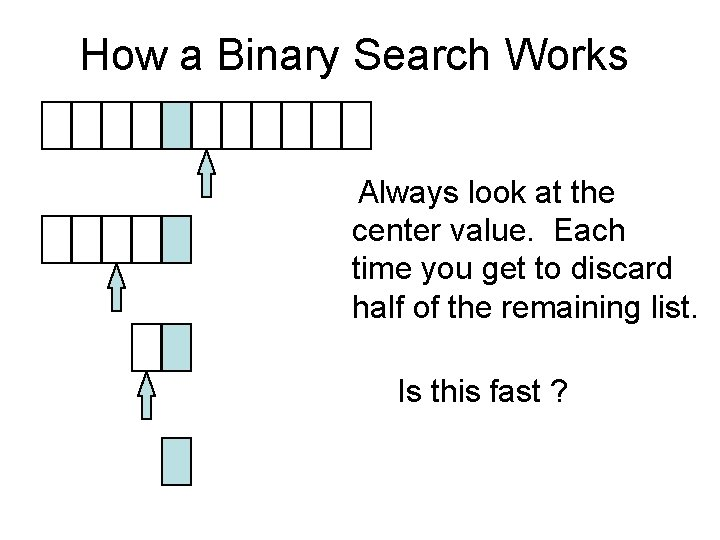How a Binary Search Works Always look at the center value. Each time you get to discard half of the remaining list. Is this fast ?How Fast is a Binary Search? Worst case: 11 items in the list took 4 tries Worst case: 32 items… 1 st try - list has 16 items 2 nd try - list has 8 items 3 rd try - list has 4 items 4 th try - list has 2 items 5 th try - list has 1 item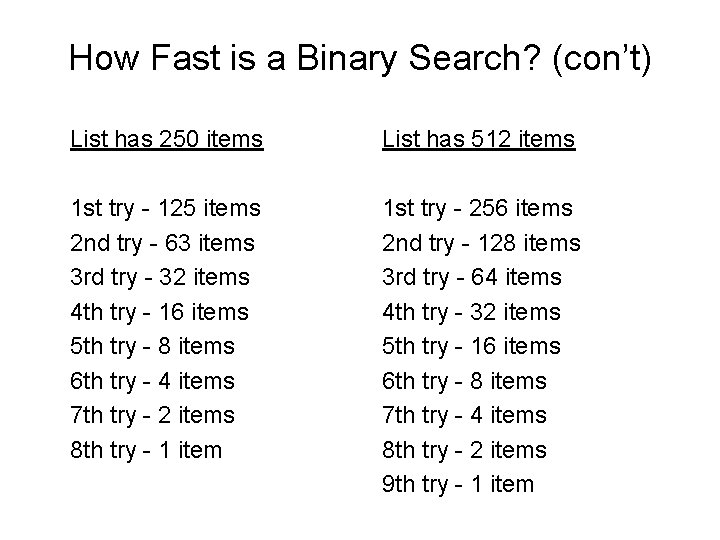How Fast is a Binary Search? (con’t) List has 250 items List has 512 items 1 st try - 125 items 2 nd try - 63 items 3 rd try - 32 items 4 th try - 16 items 5 th try - 8 items 6 th try - 4 items 7 th try - 2 items 8 th try - 1 item 1 st try - 256 items 2 nd try - 128 items 3 rd try - 64 items 4 th try - 32 items 5 th try - 16 items 6 th try - 8 items 7 th try - 4 items 8 th try - 2 items 9 th try - 1 item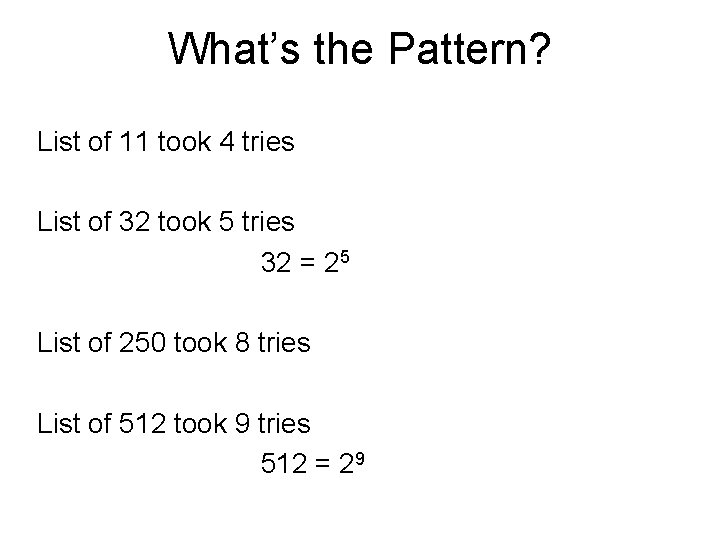What’s the Pattern? List of 11 took 4 tries List of 32 took 5 tries 32 = 25 List of 250 took 8 tries List of 512 took 9 tries 512 = 29A Very Fast Algorithm! How long (worst case) will it take to find an item in a list 30, 000 items long? 210 = 1024 211 = 2048 212 = 4096 213 = 8192 214 = 16384 215 = 32768 So, it will take only 15 tries!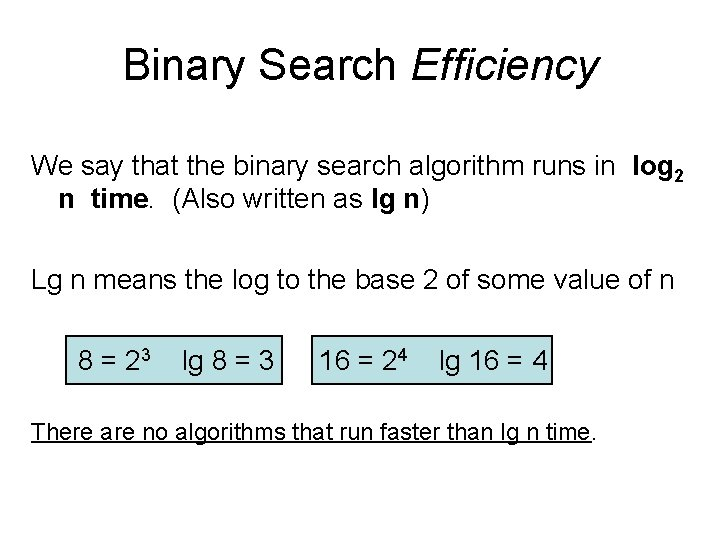Binary Search Efficiency We say that the binary search algorithm runs in log 2 n time. (Also written as lg n) Lg n means the log to the base 2 of some value of n 8 = 23 lg 8 = 3 16 = 24 lg 16 = 4 There are no algorithms that run faster than lg n time.Big O Notation • Comparing algorithms “Analysis of Algorithms” Order of Magnitude - O(…)How do we compare algorithms? • Comparing execution time Varies depending on computer. • Counting instructions Varies depending on language & computer (machine code dependent). • Count passes through critical sections of code more standardized. (usually loops!)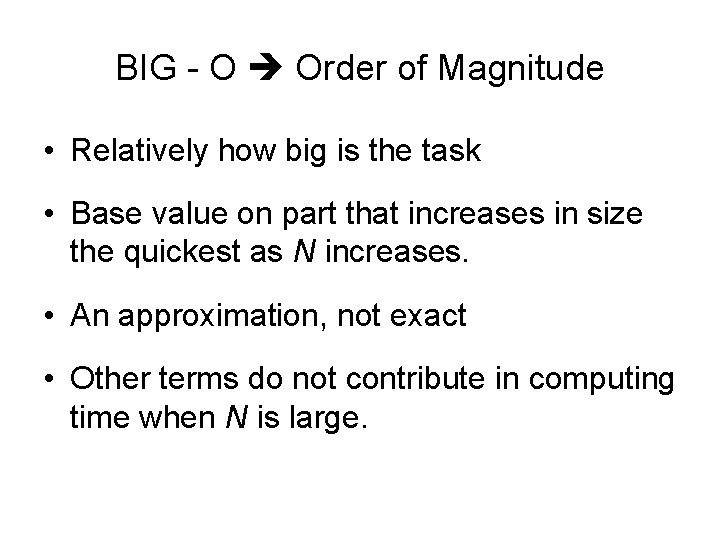BIG - O Order of Magnitude • Relatively how big is the task • Base value on part that increases in size the quickest as N increases. • An approximation, not exact • Other terms do not contribute in computing time when N is large.Common Orders of magnitude O(1) - bounded time (# elements doesn’t matter) (unsorted Insert( )) O(N) - linear time (unsorted Is. Present( ), Delete( ), sorted Insert( )) (Amount of work is some constant times the number of elements) O(log 2 N) - logarithmic time - problem splits with each pass (binary search) O(N log 2 N) - N log 2 N time - Applying a logarithmic algorithm N times. (good sorts) O(N 2) - quadratic time - Applying a linear algorithm N times (selection sort, insertion sort)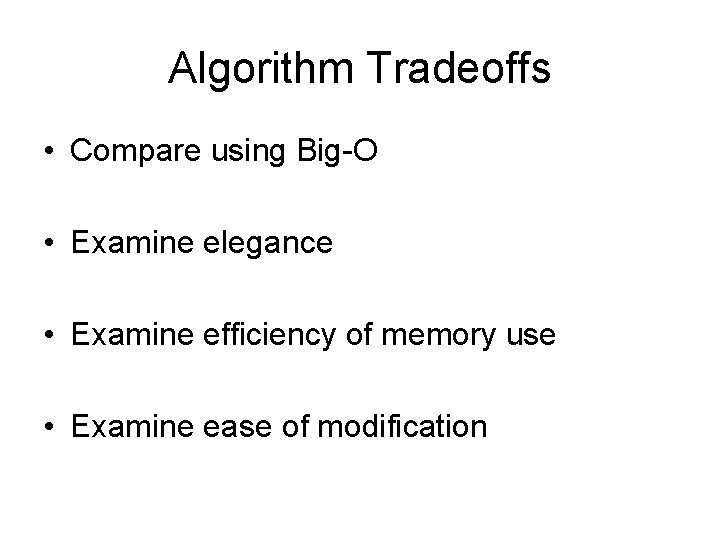Algorithm Tradeoffs • Compare using Big-O • Examine elegance • Examine efficiency of memory use • Examine ease of modification